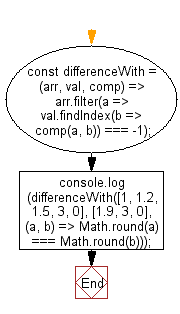# JavaScript: Filter out all values from an array for which the comparator function does not return true

## JavaScript fundamental (ES6 Syntax): Exercise-74 with Solution

Write a JavaScript program to filter out all values from an array for which the comparator function does not return true.

• Use Array.prototype.filter() and Array.prototype.findIndex() to find the appropriate values.
• Omit the last argument, comp, to use a default strict equality comparator.

Sample Solution:

JavaScript Code:

``````//#Source https://bit.ly/2neWfJ2
const differenceWith = (arr, val, comp) => arr.filter(a => val.findIndex(b => comp(a, b)) === -1);
console.log(differenceWith([1, 1.2, 1.5, 3, 0], [1.9, 3, 0], (a, b) => Math.round(a) === Math.round(b)));
```
```

Sample Output:

```[1,1.2]
```

Flowchart:Live Demo:

See the Pen javascript-basic-exercise-74-1 by w3resource (@w3resource) on CodePen.

Improve this sample solution and post your code through Disqus

What is the difficulty level of this exercise?

Test your Programming skills with w3resource's quiz.

﻿

## JavaScript: Tips of the Day

Returns an array of n-tuples of consecutive elements

Example:

```const tips_arr = (n, arr) =>  n > arr.length ? [] : arr.slice(n - 1).map((v, i) =>[...arr.slice(i, i + n - 1), v]);
console.log(tips_arr(2, [1, 2, 3, 4, 5]));
console.log(tips_arr(3, [1, 2, 3, 4, 5]));
console.log(tips_arr(5, [1, 2, 3, 4]));
```

Output:

```[[1, 2], [2, 3], [3, 4], [4, 5]]
[[1, 2, 3], [2, 3, 4], [3, 4, 5]]
[]
```